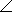# Electrical Engineering - RC Circuits

### Exercise :: RC Circuits - General Questions

11.

What is the value of the hypotenuse of a right triangle whose sides are 12 and 18?

 A. 4.24 B. 3.46 C. 2.16 D. 21.63

Answer: Option D

Explanation:

No answer description available for this question. Let us discuss.

12.

The complex number 6 + j6 is equivalent to

 A. 645° B. 3645° C. 8.4845° D. 8.4890°

Answer: Option C

Explanation:

No answer description available for this question. Let us discuss.

13.

In a parallel RC circuit, there is 100 mA through the resistive branch and 100 mA through the capacitive branch. The total rms current is

 A. 200 mA B. 100 mA C. 282 mA D. 141 mA

Answer: Option D

Explanation:

No answer description available for this question. Let us discuss.

14.

An ac circuit consists of a resistor and a capacitor. To increase the phase angle above 45°, the following condition must exist:

 A. R = XC B. R > XC C. R < XC D. R = 5XC

Answer: Option C

Explanation:

No answer description available for this question. Let us discuss.

15.

What is the angular difference between +j4 and –j4?

 A. 30° B. 90° C. 180° D. 270°

Answer: Option C

Explanation:

No answer description available for this question. Let us discuss.

#### Current Affairs 2021

Interview Questions and Answers